## Biophysics and Beer# Orienting

## Orienting¶

We're in New Zealand for a semester. Recently, Ash Patea was telling us some Māori lore about local mountains. We're based in Whanganui, learning about the mountains Tongariro, Taranaki, and Ruapehu, all of which are to our north. Ash starts all of these sessions off by telling us that we're learning his iwi's stories, but that they're not the only stories. That other stories are different, and none is "right." We then get to the part where we learn that the name "Tongariro" tells you it's a mountain to the south, and Ash says that's how they know the story we're learning isn't their story, and all of a sudden I have to write a little math post.

I don't want to spoil the punchline too much (yes I do), but there's a really neat connection between Ash's language and mathematics, about how you orient yourself in the world, and about how things can't always be consistent.

Math time. A "chart" is essentially your local set of coordinates, or how you tell yourself how to move around in the world. You can think of it like a street map in a city, or the idea of north, south, east, and west.

In :
makeone()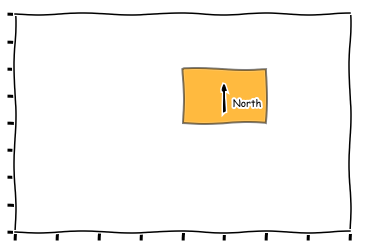You could describe different regions with different charts. You need to "glue" them together in the areas where they overlap.

In :
makethree()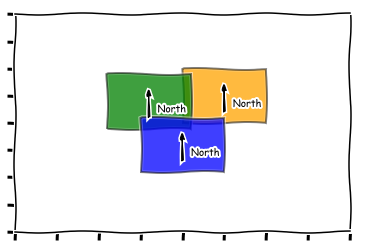When you glue all of those charts together to cover an object, the result is called an "atlas"

In :
makelots()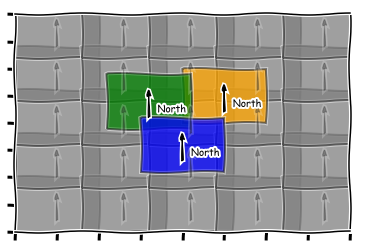So, obviously, the connection I want point out is that each of these charts is like the stories of an individual iwi. They tell that iwi how to understand the world; how to get around in the world; how to orient themselves in the world. Understanding the local map tells you where you are; and knowing how to glue those local maps together tells you how to get around.

Sometimes, you can glue everything together into one consistent set of coordinates. Like in the above pictures, we could just have called the same direction "north" everywhere. Or, maybe every iwi has the same stories. Back to math language, maybe you can just have one chart to make up your whole atlas.

#### But the thing that really struck me is that sometimes you can't do that!¶

The most common example on the math side of things is coordinates on a sphere. You already know this: you know how latitude and longitude don't really work on at the North Pole or the South Pole? Well, it turns out that's because you can't cover a sphere with one chart!

Here's one example

In :
make_half_sphere_chart()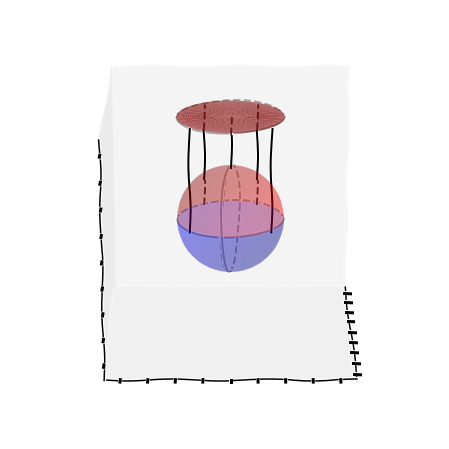where you can see a chart in red mapping the top half of the sphere ... but obviously not the bottom half. Like I said, though, it turns out that you simply can't have one chart that covers the whole sphere. Kind of like how, when we say "north" we don't mean the direction that goes through the inside of the earth straight towards the North Pole. We mean the direction we'd draw on the ground. And that direction is pointing somewhere different for someone in New Zealand vs. someone in the US.

In :
make_north_example()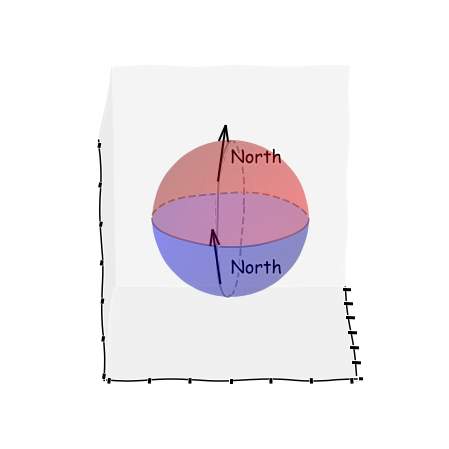This turns out to be related to the Hairy Ball Theorem. That one says that, if you have a ball covered with hair (like my head, or a basketball), you can't comb it without at least one cowlick. To be specific, the hairs are pointing in some direction along the head, and you're fundamentally stuck with at least one place where your local directions don't agree!

#### Anyway, that's a bit of a digression. Back to what we were learning from Ash.¶

We were learning Māori legends, and they’re different in different geographical regions. They often conflict if you get far enough away. The specific example that came up was talking about stories of the histories of the mountains nearby. The story Ash told us was about a mountain called Tongariro. Skipping lots of details, but Tongariro is a mountain to our north. But if you understand the Māori meaning of "Tongariro" it tells you that the mountain is to the south!

Back to the math language, you're clearly looking at two different charts, and they don't match up! You have to be very careful about how you translate ("glue") between them, because they don't agree.

### I think that's the coolest thing! That the way Māori stitch together culture is exactly the way mathematicians stitch together coordinates!¶

You just have to understand that they're different in different places, and you have to use that context to understand what's going on.

Pākehā (non-Māori folks) often try to say "this is THE one Māori legend" i.e. to impose some global coordinates. A really powerful example of this is the Moriori.

In the early 19th century, a couple of pākehā ethnologists were trying to knit together Māori stories into a global whole. They kept finding pieces that didn't match up. Often, these were stories of people who had been in Aotearoa/New Zealand before. It seems clear now that these were simply stories of other Māori groups, and stories of fighting between different iwi. The ethnologists, however, invented the "Moriori myth": they claimed that the Moriori were here when Māori came to Aotearoa/New Zealand. The truth is that the Māori came to New Zealand, and then some of them left to go to the Chatham Islands. The group that left became the Moriori.

The Moriori myth led to direct material harm: the idea that "the Māori did it to the Moriori first" was used by colonizers to justify ignoring the Treaty of Waitangi for a hundred years!

Back to the math for a second. It also turns out that, for most surfaces, you can't have one global set of coordinates. You have to understand the local charts, and then you have to understand how to glue them together into an atlas. Maybe we should take something socially from that.

And maybe I had to come all the way to the other side of the globe, to Aotearoa/New Zealand to figure out how to start piecing together local views of the world, to glue my charts into an atlas without just stretching one chart past its relevance.

Edits: I made my own versions of all of the images, made an image for the "north" example, cleaned up some of the language, added a bit more about Moriori, and moved the "orientable" punchline to the endnotes.

Endnotes:
(1) Māori talk about how these stories are how they orient themselves in the world. "Orient" has a meaning in math as well, and it essentially means that you don't just know how to define north/south/east/west, you also know how to define "up" and "down." A non-orientable surface is effectively one where you can have local definitions of "up" and "down" everywhere, but there is fundamentally no way to define a global coordinate system, to define "up" and "down."

And it turns out that most surfaces are non-orientable. Again, maybe we should take something from that.
(2) For the math folks, when I say surface above, I really mean Manifold.
(3) We learned the Moriori history from Jillian Wychel and David James, and I don't have a great website/link for them. The history of Māori and Moriori is not a simple one, and there's a lot of ugliness, especially as the Moriori were almost completely wiped out in the 1800s.
(4) If you're curious about the code that produced those plots, here it is!

In :
from matplotlib.patches import Rectangle
from matplotlib.path import Path
import matplotlib.patches as patches
import numpy as np, matplotlib.pyplot as plt
from mpl_toolkits.mplot3d import Axes3D
plt.xkcd()

def plt1(ax,offsetx=0,offsety=0,color='orange',text=True):
# Mostly taken from
# https://matplotlib.org/users/path_tutorial.html
verts = [
(0. + offsetx, 0. + offsety), # left, bottom
(0. + offsetx, 1. + offsety), # left, top
(1. + offsetx, 1. + offsety), # right, top
(1. + offsetx, 0. + offsety), # right, bottom
(0. + offsetx, 0. + offsety), # ignored
]

codes = [Path.MOVETO,
Path.LINETO,
Path.LINETO,
Path.LINETO,
Path.CLOSEPOLY,
]

path = Path(verts, codes)

patch = patches.PathPatch(path, facecolor=color, lw=2, alpha=0.5)

#arrow( x, y, dx, dy, **kwargs )
if text:
alpha=1
else:
alpha=0.2
plt.arrow( 0.5 + offsetx, 0.2 + offsety, 0.0, 0.4, fc="k", ec="k",head_width=0.05, head_length=0.1,alpha=alpha)
if text:
plt.text(0.6 + offsetx,0.3 + offsety,'North')
ax.set_xlim(-2,2)
ax.set_ylim(-2,2)
ax.set_yticklabels([])
ax.set_xticklabels([])

def makeone():
fig = plt.figure()

plt1(ax)

def makethree():
fig = plt.figure()

plt1(ax)
plt1(ax,-0.9,-0.1,'green')
plt1(ax,-0.5,-0.9,'blue')

def makelots():
fig = plt.figure()

for i in np.arange(-2,2,.8):
for j in np.arange(-2,2,.8):
plt1(ax,i,j,'grey',text=False)

plt1(ax)
plt1(ax,-0.9,-0.1,'green')
plt1(ax,-0.5,-0.9,'blue')

def plot_top(ax,n_theta,n_phi,r,fixz=False,alpha=0.5):
# Largely taken from
# https://stackoverflow.com/questions/41105754/heat-map-half-sphere-plot

#theta inclination angle
#phi azimuthal angle

theta, phi = np.mgrid[0.0:0.5*np.pi:n_theta*1j, 0.0:2.0*np.pi:n_phi*1j]

x = r*np.sin(theta)*np.cos(phi)
y = r*np.sin(theta)*np.sin(phi)
z = r*np.cos(theta)
if fixz:
z = np.ones_like(z)*2

ax.plot_surface(
x,y,z,  rstride=1, cstride=1,
color='red',
alpha=alpha, linewidth=1)

def plot_bottom(ax,n_theta,n_phi,r,alpha=0.5):
# Largely taken from
# https://stackoverflow.com/questions/41105754/heat-map-half-sphere-plot

#theta inclination angle
#phi azimuthal angle

theta, phi = np.mgrid[0.0:0.5*np.pi:n_theta*1j, 0.0:2.0*np.pi:n_phi*1j]

x = r*np.sin(theta)*np.cos(phi)
y = r*np.sin(theta)*np.sin(phi)
z = -r*np.cos(theta)

ax.plot_surface(
x,y,z,  rstride=1, cstride=1,
color='blue',
alpha=alpha, linewidth=1)

def plot_circles(ax,includetop=True,color='k'):
# Largely taken from
# https://stackoverflow.com/questions/32424670/python-matplotlib-drawing-3d-sphere-with-circumferences
u = np.linspace(0, 2 * np.pi, 100)
v = np.linspace(0, np.pi, 100)

elev = 10.0
rot = 80.0 / 180 * np.pi

#calculate vectors for "vertical" circle
a = np.array([-np.sin(elev / 180 * np.pi), 0, np.cos(elev / 180 * np.pi)])
b = np.array([0, 1, 0])
b = b * np.cos(rot) + np.cross(a, b) * np.sin(rot) + a * np.dot(a, b) * (1 - np.cos(rot))
horiz_front = np.linspace(0, np.pi, 100)
ax.plot(np.sin(u),np.cos(u),0,color=color, linestyle = 'dashed')
ax.plot(np.sin(horiz_front),np.cos(horiz_front),0,color=color)

if includetop:
ax.plot(np.sin(u),np.cos(u),2.0,color=color, linestyle = 'dashed')
ax.plot(np.sin(horiz_front),np.cos(horiz_front),2.0,color=color)

vert_front = np.linspace(np.pi / 2, 3 * np.pi / 2, 100)
ax.plot(a * np.sin(u) + b * np.cos(u), b * np.cos(u), a * np.sin(u) + b * np.cos(u),
color=color, linestyle = 'dashed')
ax.plot(a * np.sin(vert_front) + b * np.cos(vert_front),
b * np.cos(vert_front), a * np.sin(vert_front) + b * np.cos(vert_front),
color=color)

def plot_lines(ax):
ax.plot(xs=[0.75,.75],ys=[-0.75,-0.75],zs=[0,2],color='k',alpha=1.0)
ax.plot(xs=[0.75,.75],ys=[0.75,0.75],zs=[0,2],color='k',alpha=1.0)

ax.plot(xs=[0,0],ys=[0,0],zs=[1,1.65],color='k')
ax.plot(xs=[0,0],ys=[0,0],zs=[1.65,2.0],color='k',linestyle='dashed',alpha=0.75)

ax.plot(xs=[-0.75,-.75],ys=[-0.5,-0.5],zs=[0.5,1.5],color='k',alpha=1.0)
ax.plot(xs=[-0.75,-.75],ys=[-0.5,-0.5],zs=[1.5,2.0],color='k',linestyle='dashed',alpha=0.75)
ax.plot(xs=[-0.75,-.75],ys=[-0.5,-0.5],zs=[0,0.5],color='k',linestyle='dashed',alpha=0.75)

ax.plot(xs=[-0.75,-.75],ys=[0.5,0.5],zs=[0.5,1.5],color='k',alpha=1.0)
ax.plot(xs=[-0.75,-.75],ys=[0.5,0.5],zs=[1.5,2.0],color='k',linestyle='dashed',alpha=0.75)
ax.plot(xs=[-0.75,-.75],ys=[0.5,0.5],zs=[0,0.5],color='k',linestyle='dashed',alpha=0.75)

def make_half_sphere_chart():
fig = plt.figure(figsize=(10, 8))

plot_top(ax,30,30,1,alpha=0.25)
plot_bottom(ax,30,30,1,alpha=0.25)
plot_circles(ax,color='darkgrey')
plot_top(ax,30,30,1,fixz=True,alpha=0.7)
plot_lines(ax)

ax.set_xlim([-2.2,2.2])
ax.set_ylim([-2.2,2.2])
ax.set_zlim([-2.2,2.2])

ax.set_aspect('equal')
ax.view_init(elev = 20, azim = 0)
ax.set_yticklabels([])
ax.set_xticklabels([])
ax.set_zticklabels([])

def make_north_example():
fig = plt.figure(figsize=(10, 8))

plot_top(ax,30,30,1,alpha=0.25)
plot_bottom(ax,30,30,1,alpha=0.25)
plot_circles(ax,includetop=False,color='darkgrey')

offsetx = 0
offsety = 0
alpha=1.0
# These arrows aren't mathematically correct ... but they look reasonable!
ax.quiver(,[-.13],[-0.9],[ 0],[-.1],[.75],color='k')
ax.text(0,0,-.75,'North',fontsize=18)

ax.quiver(,[-.16],[0.5], ,[.1],[.75],color='k')
ax.text(0,0,.75,'North',fontsize=18)

ax.set_xlim([-1.5,1.5])
ax.set_ylim([-1.5,1.5])
ax.set_zlim([-1.5,1.5])

ax.set_aspect('equal')
ax.view_init(elev = 20, azim = 0)
ax.set_yticklabels([])
ax.set_xticklabels([])
ax.set_zticklabels([])